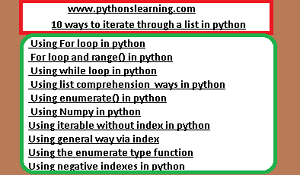# 10 ways to iterate through a list in python

The list is similar to array in other languages except for python, which provides the extra benefit of being dynamic in size nature. In Python, the list is a type of  Data Structures, which is used to store different and multiple kinds of data at the same time.10 ways to iterate through a list in python

## There are many ways to iterate over a list in Python language. Let’s see all different ways in python:

1.  Using For loop in python
2.  For loop and range() in python
3.  Using while loop in python
4.  Using list comprehension  ways in python
5.  Using enumerate() in python
6.  Using Numpy in python
7. Using iterable without index in python
8. Using general way via index
9. Using the enumerate type function
10. Using negative indexes in python

### Method #1: Using For loop in python

```

# Python3 code to iterate over a list in python

list = [1, 3, 5, 8, 9]

# Using for loop

for n in list:

print(n)

```
Output:

```

1

3

5

8

9

```

#### Method #2: For loop and range() in python

In case we want to use the traditional For loop and range() in python which iterates from number x to number y.

```

# Python3 code to iterate over a list in python

num = [1, 3, 5, 8, 9]

# getting length of list in python

length = len(num)

# How to Iterating the index

for i in range(length):

print(list[i])

```
learn pandas in python
Output:

```

1

3

5

8

9

```
Method #3: Using while loop in python

```

# Python3 code to iterate over a list in python

num = [1, 3, 5, 8, 9]

# Getting length of list in python

length = len(num)

i = 0

# Iterating using while loop in python

while i < length:

print(num[i])

i += 1

```
Output:

```

1

3

5

8

9

```
Method #4: Using list comprehension  ways in python

```

# Python3 code to iterate over a list in python

num = [1, 3, 5, 8, 9]

# Using list comprehension in python

[print(i) for i in num]

```
Output:

```

1

3

5

8

9

```
Method #5: Using enumerate() in python
suppose if we want to convert the list of set into an iterable list of tuples, then you can use the enumerate() function in python.

```

# Python3 code to iterate over a list in python

num = [1, 3, 5, 8, 9]

# Using enumerate() function in python

for i, val in enumerate(num):

print (i, ",",val)

```
Output:

```

0 , 1

1 , 3

2 , 5

3 , 8

4 , 9

```
Method #6: Using Numpy in python
If in code we provide n-dimensional lists, then it is sometimes better to use an external library (NumPy)
filter_none

```

# Python program for using numpy func.

# iterating over array in python

import numpy as geek

z = z.reshape(3, 3)

# iterating an array in python

for y in geek.nditer(z):

print(y)

```
Output:

```

0

1

2

3

4

5

6

7

8

```

Method 7# − Using iterable without index in python
Example
```

sach_list = ['p','y','t','h','o','s','l','e','a','r','n','i','n','g']

# Iterate over the list in python

for value in sach_list:

print(value, end='')

output:

pythonslearning

```

Method 8# − Using general way via index
Example
```

sach_list = ['p','y','t','h','o','s','l','e','a','r','n','i','n','g']

# Iterate over the list in python

for value in range(0,len(sach_list)):

print(sach_list[value], end='')

output:

pythonslearning

```

Method 9# − Using the enumerate type function
Example
```

sach_list = ['p','y','t','h','o','s','l','e','a','r','n','i','n','g']

# Iterate over the list in python

for value,char in enumerate(sach_list):

print(char, end='')

output:

pythonslearning

```

Method 10# − Using negative indexes in python
Example
```

sach_list = ['p','y','t','h','o','s','l','e','a','r','n','i','n','g']

output:

pythonslearning

```
Summary:
If you want to learn linear regression in python then click on it.

Tags:
10 ways to iterate through a list in python
BEST OF LUCK!!!